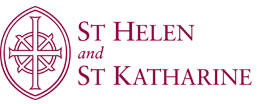Mathematics Department Mathematics The following is guidance from the exam boards using QCDA guidance. A* will be awarded at A level (but not at AS) to students cashing-in from Summer 2010 onwards. The general principle is that achievement at A2 should be recognised and so an A* will be awarded to students who achieve a grade A overall and 90% or more of the UMS on the A2 units (as an aggregate, not on each separate A2 unit). However, since some Mathematics combinations have three A2 units and some have two A2 units, the A* will be awarded to students who have achieved a grade A overall (480 UMS or more) and 180 UMS or more on the total of their C3 and C4 units. There will be some cases where a student with a high UMS score is awarded a grade A and a student with a relatively low UMS score is awarded a grade A* – but the A* is principally to do with recognising performance on C3 and C4. In the most extreme cases, an A* grade could be awarded to a candidate with 480 UMS and an A grade to a candidate with 579 UMS. Examples: C1 = 90, C2 = 79, C3 = 95, C4 = 94, M1 = 87, M2 = 89Total = 534 UMS, grade A* awarded C1 = 70, C2 = 70, C3 = 93, C4 = 94, M1 = 77, S1 = 78Total = 482 UMS, grade A* awarded C1 = 95, C2 = 98, C3 = 92, C4 = 87, D1 = 87, D2 = 89Total = 548 UMS, grade A awarded C1 = 90, C2 = 92, C3 = 91, C4 = 92, M1 = 57, D1 = 57Total = 479 UMS, grade B awarded Further Mathematics For Further Mathematics, A* will be awarded to students who have achieved a grade A overall (480 UMS or more) and 270 UMS or more on their best three A2 units (whether pure or applications units). Examples: FP1 = 93, FP2 = 91, S1 = 95, S2 = 91, D1 = 30, D2 = 89Total 489 UMS, grade A* awarded FP1 = 80, FP2 = 86, M2 = 94, M3 = 85, M4 = 88, M5 = 89Total 522 UMS, grade A* awarded FP1 = 80, FP2 = 86, M2 = 94, M3 = 85, M4 = 86, M5 = 89Total 520 UMS, grade A awarded As you can see, there will be some cases where a student with a high UMS score is awarded a grade A and a student with a relatively low UMS score is awarded a grade A* – but it’s to do with recognising performance on C3 and C4. Re-sits will be acceptable; when a student has his of her Mathematics grade awarded from summer 2010 onwards, it will be the highest relevant UMS marks in the bank that will be used to make the calculation. Article from the BBC about A* grades. Last updated 8.35pm, 28/05/2010St Helen and St Katharine Trust, Company No. 1710695, Registered in England, at Faringdon Road, Abingdon, OX14 1BE